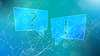TechTopics No. 74

Heat generation estimation for type GM-SG or GM-SG-AR switchgear (up to 15 kV)

We are often asked to provide estimated heat generation data for our equipment. This issue of TechTopics provides information that allows calculation of approximate heat generated by the type GM-SG or GM-SG-AR switchgear under assumed loading conditions.

The heat generation data given in the table is based on full-rated continuous current. Actual heat generation calculations must take into account the true loading of the equipment.

The amount of heat generated is related to the square of the current, so a circuit breaker operating at one-half rated current will have heat generation only one-quarter of that at full-rated continuous current. Because the effect of the square relationship is very significant, it is overly conservative to estimate heat generation based on the assumption that all sections and all circuit breakers each carry their rated continuous current at all times.

Air-conditioning systems sized based upon such estimates will be much larger than the real operating conditions will require. To estimate the heat generated under actual loading conditions, determine the component heat generation for each of the components indicated in Table 1. Estimated heat generation for circuit breakers should be adjusted for actual loading based on the ratio of the squares of the actual current and the rated current.

Table 1: Approximate full-load heat generation (in watts (W)) for type GM-SG or GM-SG-AR switchgear (up to 15 kV)
Description

Continuous current circuit breaker (rows 1-3) or main bus (row 4)
Category
Related current
1,200 A
2,000 A
3,000 A
4,000 A
Category
Actual current
1,200 A
2,000 A
3,000 A
4,000 A
Circuit breaker cell with circuit breaker

576 W
888 W
1,391 W
2,473 W
Vertical section with main bus

154 W
190 W
250 W
380 W
Space heaters per vertical section

400 W
400 W
400 W
400 W
Voltage transformer (VT) rollout

50 W
50 W
50 W
50 W
Control power transformer (CPT) (drawout or stationary)

4% of CPT kVA rating
4% of CPT kVA rating
4% of CPT kVA rating
4% of CPT kVA rating
Relaying and instrumentation per circuit breaker cell
Microprocessor type
50 W
50 W
50 W
50 W
Relaying and instrumentation per circuit breaker cell
Electromechanical non-complex
100 W
100 W
100 W
100 W
Relaying and instrumentation per circuit breaker cell
Electromechanical complex
200 W to 300 W
200 W to 300 W
200 W to 300 W
200 W to 300 W

To be precise, this adjustment should also be made for the actual current loading of the main bus for each individual vertical section, but this is frequently ignored in the interest of simplification. Instead, the main circuit breaker loading is usually assumed to be equal to the main bus loading in all vertical sections.

Example: Assume a lineup with three vertical sections, one 2,000 A main circuit breaker (loaded to 1,400 A), three

1,200 A feeder circuit breakers (loading 250 A, 600 A and 550 A), 2,000 A main bus and space heaters. The lineup includes one VT rollout, one 10 kVA CPT and microprocessor relaying, and instrumentation. The calculations would be as described in Table 2.

If true loading were not considered (for example, all calculations performed on the basis of full-rated current), the calculations would yield a heat generation of 5,036 W or about 176 percent of the “real” heat generation.

Table 2: Calculations
Category
Description
Heat generation
A
2,000 A circuit breaker at 1,400 A = 888 x (1,400/2,000)2
435 W

A
1,200 A circuit breaker at 250 A = 576 x (250/1,200)2
25 W

A
1,200 A circuit breaker at 600 A = 576 x (600/1,200)2 =
144 W

A
1,200 A circuit breaker at 550 A = 576 x (550/1,200)2 =
121 W

A
Total heat generation for circuit breaker cells

725 W
B
Vertical sections with 2,000 A bus at 1,400 A = 3 x 190 x (1,400/2,000)2

279 W
C
Space heaters for three vertical sections = 3 x 400 =

1,200 W
D
VT rollout = 1 x 50 =

50 W
E
CPT = 1 x 4% x 10 kVA =

400 W
F
Relaying and instrumentation = 4 x 50 =

200 W
Total

2,854 W

Notes on assumptions:

1. Space heaters, when provided, are not normally controlled by a thermostat. Hence, their load is represented as a continuous load. The purpose of space heaters is to prevent condensation, and this is not limited by the absolute temperature. Even when a thermostat is used to control the heaters, it is set to shut the heaters off at a temperature of approximately 110 ºF. Therefore, in an air-conditioned room, the heaters would be energized continuously.
2. Heat generated by current transformers is ignored as it is usually insignificant and varies according to the CT ratio as well as the loading.
3. The CPT heat generation estimate is very conservative and assumes the CPT is operated at full-rated capacity. If normal loading is at less than full rating, heat generation may be adjusted by the square of the percent loading.
4. Relaying and instrumentation heat generation estimates are very approximate and are normally estimated on the basis of the number of circuit breaker cells. Extensive relaying and instrumentation may warrant additional conservatism in the estimation of associated heat generation.
5. Conversion factor: watts x 3.415179 = BTU/hour.